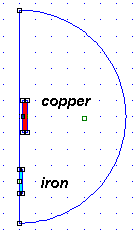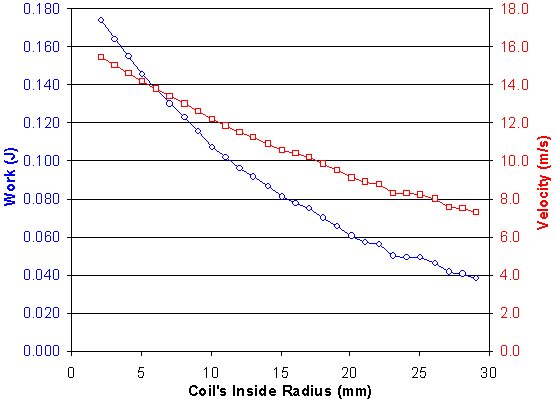# FEMM - Coil Diameter

What coil diameter works best? Is there any advantage in a very small or large inside radius? Let's use FEMM to compare the forces from various coils that differ only in their opening size.

So far, we've found efficiency does not depend on the projectile's thickness. And we found the best projectile is about 25% shorter than the coil's length. Now let's see if how the coil's diameter affects efficiency.

We'll use the same 20mm long coil with 3mm thick windings. We'll use the best projectile so far, which is 15mm long and has a 2mm radius. All my FEMM files are provided again, in the hopes that you will check my results and also experiment with your own variations.

## FEMM Model for ProjectilesThe general wisdom has been that the coil should have as small an opening as possible. In theory it results in higher magnetic field density, since it applies the emf to a smaller cross-sectional area. Let's see if this is true.

We'll arbitrarily choose a solid iron cylinder of 2mm radius. In a previous web page we found the shape of the projectile doesn't matter, and this shape is easy to model. For instructions to run FEMM with this model, see my previous page.

Let's use Lua scripting again, and this time we'll try a range of coil aperture sizes.A few trial runs with small-mouthed coils hinted at a linear relationship, so let's try a very large range of sizes. I want to examine coils from 2.1mm inside radius (barely larger than the projectile) to 32.1mm radius (a really huge opening), in 1mm steps. This can all be done in one simulation.

I adjusted mesh size to speed up the simulation. It turns out the maximum mesh sizes were 0.4mm in iron and copper, and 1mm in air. My goal was that the results should be within 1% of that from tighter mesh sizes. The inaccuracies tend to occur at the larger coil sizes such as 30mm inside radius. As a result, it took "only" 2hr 20min to run this FEMM analysis, using a Gateway 1.4 GHz Pentium 4. Admit it, this particular problem takes a lot of computation!

## Assumptions

Like my other FEMM models, this is a fairly simplistic model. However, it is a reasonable approach:

• frequency is d.c. (no eddy currents)
• velocity is zero (every point is simulated at steady-state with no motion)
• projectile is M-19 steel (nonlinear BH curve)
• steady coil current (not a capacitive-discharge system)
• no losses due to friction (don't we wish!)

## Pre-processor Lua Program

 coil_diameter_pre.lua ```outfile = "coil_diameter_results.txt" handle=openfile(outfile,"a") write(handle, "\nComparing various coil diameters \n") write(handle, "Projectile is 15mm long and 2mm radius\n") write(handle, "Coil is 20mm long with constant cross-section\n") write(handle, "Coil inside radius varies:\n") write(handle, " 2.1, 3.1, 4.1, ..., 30.1mm\n") write(handle, "To import in Excel:\n") write(handle, " Data - Get External Data - Import text file\n") write(handle, " then choose the file written by this program\n") write(handle, " and click Finish.\n") write(handle, "This data file is tab delimited\n") write(handle, " (Excel's default import format)\n") closefile(handle) -- Save the model in temporary file just to be safe save_femm_file("temp.fem") -- Set edit mode for selecting and moving projectile (and coil) -- using simple commands seteditmode("group") -- This is how much to increase the coil size between each trial increment = 1.0 for i=0,27 do -- New inner radius is starting size plus how much it has increased radius = 2.1 + i*increment -- Announce the coil dimensions in the results file handle=openfile(outfile,"a") write(handle, "\n") write(handle, "Coil inside radius = ", radius, " mm\n") write(handle, "Coil outside radius = ", radius+3.0, " mm\n") write(handle, "\n") closefile(handle) -- Move the projectile through 40 positions along the axis -- and find the force at each spot for n=1,40 do showmesh() analyse() -- Pass current position into post-processor handle=openfile("tempfile","w") pos = n-41 write(handle, pos) closefile(handle) runpost("coil_diameter_post.lua") -- Nudge projectile another millimeter upward into coil selectgroup(1) move_translate(0,1) end -- Move projectile back down to starting position outside coil selectgroup(1) move_translate(0,-40) -- Increase the coil's inner and outer radius selectgroup(2) move_translate(increment,0) end messagebox("All done!") ```

## Post-processor Lua Program

 coil_diameter_post.lua ```outfile = "coil_diameter_results.txt" -- Read position of projectile's centerpoint handle = openfile("tempfile","r") position = read(handle,"*n") closefile(handle) -- Obtain force on coil, and we assume the -- force on projectile is simply opposite in sign groupselectblock(2) force = -blockintegral(12) handle=openfile("coil_diameter_results.txt","a") write(handle, position, "\t", force, "\n") closefile(handle) exitpost()```

## Results

You can see the detailed Excel spreadsheet here. You can get the raw results file here as it was written by FEMM. For each coil, it computes the force on the projectile at many positions and sums the result.

This is a numerical solution to integrating force as it acts over a distance, yielding "work". We ignore friction and losses, and assume the work is converted completely into kinetic energy.

We can directly compare one coil with another, because the projectile is always the same. Our goal is to find how this projectile will behave with various coils.

## ConclusionThe graph shows how much kinetic energy the projectile will absorb from various coil sizes. It also shows the final velocity, assuming that KE = 1/2 * m * v2.

Smaller openings are better than bigger coils. In fact, smaller is always better. The graph proves that minimizing the air gap is important.

The energy transfer is not very sensitive to coil opening size. It may look like large changes in the graph, but that's because it includes truly huge coils. All the interesting figures are along the left edge of this chart. After all, nobody needs to make a coil opening more than 5mm bigger than the projectile!

For example, there is only a 5% difference in kinetic energy between a 2.1mm and 3.1mm opening. This is much smaller than expected, since the air gap is 0.1mm and 1.1mm respectively which is a big difference.

You can also see that velocity is nearly linear with respect to size of opening. I had been hoping that efficiency would explode upward with very small air gaps, but now we see that it is not critical. Actually this is a relief -- now I don't need to worry about tight sliding fit, or very thin-walled firing tubes!

The bottom line... It is good to minimize the coil's inside radius, but there is no extra benefit from really close-tolerance machining or expensive thin-walled firing tubes.

 < Previous Page 5 of 8 Next >